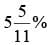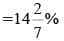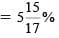# Olympiad Test: Profit And Loss - 1

## 20 Questions MCQ Test Mathematics Olympiad Class 7 | Olympiad Test: Profit And Loss - 1

Description
Attempt Olympiad Test: Profit And Loss - 1 | 20 questions in 40 minutes | Mock test for Class 7 preparation | Free important questions MCQ to study Mathematics Olympiad Class 7 for Class 7 Exam | Download free PDF with solutions
QUESTION: 1

### Alfred buys an old scooter for Rs 4700 and spends Rs 800 on its repairs. If he sells the scooter for Rs 5800, his gain percent is:

Solution:

Cost Price (C.P.) = Rs (4700 + 800)
= Rs 5500
Selling Price (S.P.) = Rs 5800
Gain = (S.P.) – (C.P.) = Rs (5800 – 5500)
= Rs 300
Gain % = (300/5500) × 100% =QUESTION: 2

### The cost price of 20 articles is the same as the selling price of x articles. If the profit is 25%, then the value of x is:

Solution:

Let C.P. of each article be Rs 1, C.P.
of x articles = Rs x
S.P. of x articles = Rs 20
Profit = Rs (20 – x)
Therefore, (20 – x)/x × 100 = 25
⇒ 2000 – 100x = 25x
125x = 2000
⇒ x = 16

QUESTION: 3

### If selling price is doubled, the profit triples. Find the profit percent.

Solution:

Let C.P. be Rs x and S.P. be Rs y.
Then, 3(y – x) = (2y – x) y = 2x
Profit = Rs (y – x) = Rs (2x – x) = Rs x
Profit % = (x/x × 100) % =100%

QUESTION: 4

In a certain store, the profit is 320% of the cost. If the cost increases by 25% but the selling price remains constant, approximately what percentage of the selling price is the profit?

Solution:

Let C.P. = Rs 100. Then, Profit = Rs 320,
S.P. = Rs  420
New C.P. = 125% of Rs 100 = Rs 125
New S.P. = Rs 420
Profit = Rs (420 – 125) = Rs 295
Required percentage = (295/420 × 100) %
= (1475/21) % = 70% (approximately).

QUESTION: 5

A vendor bought toffees at 6 for a rupee. How many for a rupee must he sell to gain 20%?

Solution:

C.P. of 6 toffees = Rs 1
S.P. of 6 toffees = 120% of Rs 1 = Rs 6/5
For Rs 6 /5, toffees sold = 6.
For Rs 1 , toffees sold =(6 × 5/6) = 5

QUESTION: 6

The percentage profit earned by selling an article for Rs 1920 is equal to the percentage loss incurred by selling the same article for Rs 1280. At what price should the article be sold to make 25% profit?

Solution:

Let C.P. be Rs x.
Then,[(1920 – x)/x] × 100
= [(x – 1280)/x] × 100
1920 – x = x – 1280
2x = 3200
x = 1600
Required S.P. = 125% of Rs 1600
= Rs (1 25/100) × 1600= Rs 2000

QUESTION: 7

A shopkeeper expects a gain of 22.5% on his cost price. If in a week, his sale was of Rs 392, what was his profit?

Solution:

C.P. = Rs [(100/122.5) × 392] = Rs 320
Profit = Rs (392 – 320) = Rs 72.

QUESTION: 8

A man buys a cycle for Rs 1400 and sells it at a loss of 15%. What is the selling price of the cycle?

Solution:

S.P. = 85% of Rs 1400 = Rs 1190

QUESTION: 9

Sam purchased 20 dozens of toys at the rate of Rs 375 per dozen. He sold each one of them at the rate of Rs 33. What was his percentage profit?

Solution:

Cost Price of 1 toy = Rs (375/12) = Rs 31.25
Selling Price of 1 toy = Rs 33
So, Gain = Rs (33 - 31.25) = Rs 1.75
Profit % = (1.75/31.25) × 100% = 5.6 %

QUESTION: 10

Some articles were bought at 6 articles for Rs 5 and sold at 5 articles for Rs 6. Gain percent is:

Solution:

Suppose, the number of articles bought
= L.C.M. of 6 and 5 = 30
C.P. of 30 articles = Rs (5/6 × 30) = Rs 25
S.P. of 30 articles = Rs (6/5 × 30) = Rs 36
Gain % = (11/25 x 100) % = 44%

QUESTION: 11

On selling 17 balls at Rs 720, there is a loss equal to the cost price of 5 balls. The cost price of a ball is:

Solution:

(C.P. of 17 balls) – (S.P. of 17 balls)
= (C.P. of 5 balls)
⇒ C.P. of 12 balls = S.P. of 17 balls
= Rs 720
⇒ C.P. of 1 ball = Rs (720/12) = Rs 60

QUESTION: 12

When a plot is sold for Rs 18,700, the owner loses 15%. At what price must that plot be sold in order to gain 15%?

Solution:

85 : 18700 = 115 : x
x = [(18700 × 115)/85] = 25300
Hence, S.P. = Rs 25,300

QUESTION: 13

100 oranges are bought at the rate of Rs 350 and sold at the rate of Rs 48 per dozen. The percentage of profit or loss is:

Solution:

C.P. of 1 orange = Rs 350/100 = Rs 3.50
S.P. of 1 orange = Rs 48/4 = Rs 12
Gain% = (0.50/3.50) x 100%QUESTION: 14

A shopkeeper sells one transistor for Rs 840 at a gain of 20% and another for Rs 960 at a loss of 4%. His total gain or loss percent is:

Solution:

C.P. of 1st transistor = Rs (100/120) × 840 = Rs 700
C.P. of 2nd transistor = Rs (100/96) × 960 = Rs 1000
So, total C.P. = Rs (700 + 1000) = Rs 1700
Total S.P. = Rs (840 + 960) = Rs 1800
Gain % = (100/1700) × 100%QUESTION: 15

A trader mixes 26 kg of rice at Rs 20 per kg with 30 kg of rice of other variety at Rs 36 per kg and sells the mixture at Rs 30 per kg. His profit percent is:

Solution:

C.P. of 56 kg rice = Rs (26 × 20 + 30 × 36)
= Rs (520 + 1080) = Rs 1600
S.P. of 56 kg rice = Rs (56 × 30) = Rs 1680
∴ Gain = (80/1600) × 100% = 5%

QUESTION: 16

A shopkeeper sells some articles at the profit of 25% on the original price. What is the exact amount of profit? To find the answer, which of the following information given in statementsI and II is/are necessary?
I. Sale price of the article
II. Number of articles sold

Solution:

Gain = 25% of C.P.
In order to find gain, we must know the sale price of each article and the number of articles sold.

QUESTION: 17

A shopkeeper sells some toys at Rs 250 each. What percent profit does he make? To find the answer, which of the following information given in statements I and II is/are necessary?
I. Number of toys sold
II. Cost price of each toy

Solution:

S.P. = Rs 250 each.
To find gain percent, we must know the C.P. of each.

QUESTION: 18

A man mixes two types of rice (X and Y) and sells the mixture at the rate of Rs 17 per kg. Find his profit percentage.
I. The rate of X is Rs 20 per kg
II. The rate of Y is Rs 13 per kg

Solution:

The ratio, in which X and Y are mixed, is not given.
So, both I and II together cannot give the answer.

QUESTION: 19

By selling a product with 20% profit, how much profit was earned?
I. The difference between cost and selling price is Rs 40.
II. The selling price is 120 percent of the cost price.

Solution:

Gain = 20%
I. Profit = (S.P.) – (C.P.) =Rs 40
Thus, I gives the answer. But, II does not give the answer.

QUESTION: 20

By selling an article what is the profit percent gained?
I. 5% discount is given on list price.
II. If discount is not given, 20% profit is gained.
III. The cost price of the articles is Rs 5000.

Solution:

I. Let the list price be Rs x.
Then, S.P. = 95% of Rs x = Rs (19x/20)
II. When S.P. = Rs x and gain = 20%.
Then, C.P. = Rs (100/120 × x) = Rs (5x/6)
∴ Gain  = (19x/20) – (5x/6) = (7x/60)
∴ Gain %  = [(7x/60) × (6/5x) × 100]% = 14%
Thus, I and II only give the answer.Use Code STAYHOME200 and get INR 200 additional OFF Use Coupon Code